Q:

# Given an integer, S represented as a string, get the sum of all possible substrings of this string.

Given an integer, S represented as a string, get the sum of all possible substrings of this string.

Input:

A string S that representing the number.

Output:

Print sum of all possible substrings as required result.

Constraints:

```1 <= T <= 100
1 <= S <= 1012
```

Example:

```Input:

1234
326

Output:
1670
395
```

Explanation:

```For the first input 1234,
All possible substrings are
1, 2, 3, 4, 12, 13, 23, 34, 123, 234, 1234
Total sum = 1 + 2 + 3 + 4 + 12 + 23 + 34 + 123 + 234 + 1234 = 1670
For the second input 326
All possible substrings are
3, 2, 6
32, 26
326
Total sum=3+2+6+32+26+326= 395
```

The solution approach is by storing the substring sums to compute the exact next substring sum

1. Create dp[n][n] to store substring sums;
2. Initialize sum=0 which will be our final result;
3. Base case computation (single length substrings),
```for i=0 to n-1,n= string length
dp[i][i]=s[i] -'0'; //s[i]-'0' gives the digit actually
sum+=dp[i][i];
end for
```
4. Till now we have computed all single digit substrings,
```for substring length,len=2 to n
for start=0 to n-len
//so basically it's the substring s[start,end]
int end=start+len-1;
dp[start][end]=dp[start][end-1]*10+s[end]-'0';
sum+=dp[start][end];
end for
end for
```
5. Sum is the final result.

All the statements are self-explanatory except the one which is the fundamental idea of the entire storing process. That is the below one,

```dp[start][end]=dp[start][end-1]*10+s[end]-'0';
```

Let's check this with an example,

```Say we are computing for string s="1234"
At some stage of computing,
Start=1, end= 3
So
Dp[start][end]=dp[start][end-1]*10+s[end]-'0'

So basically we are computing value of substring s[start..end]
with help of already computed s[start,end-1]

For this particular example
s[start..end] ="234"
s[start..end-1] ="23"

Now, dp=dp*10+'4'-'0'

So, assuming the fact that our algo is correct and thus dp[start][end-1]
has the correct value, dp[]1 would be 23 then
So,
dp=23*10+'4'-'0=234
and that's true
So, here's the main logic
Now how dp is guaranteed to be correct can be
explored if we start filling the Dp table from the base conditions?
```

Let's start for the same example

N=4 here

So, we need to fill up a 4X4 DP table,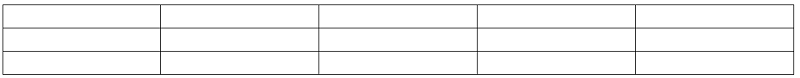After filling the base case,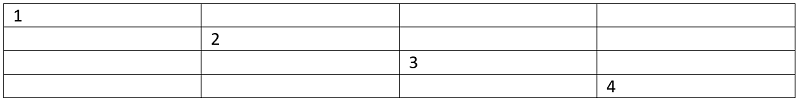Now, I am computing for len=2

Start=0, end=1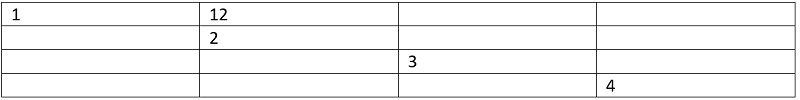Start=1, end=2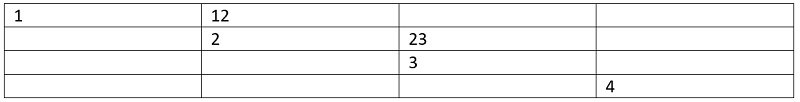Start=2, end=3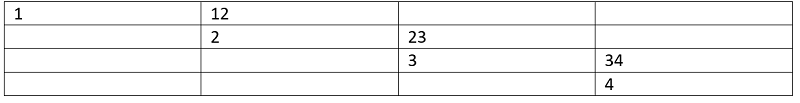For len =3

Start=0, end=2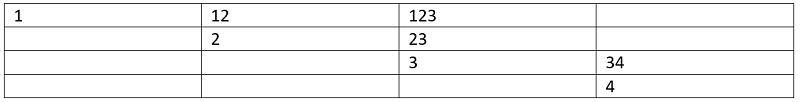Start=1, end=3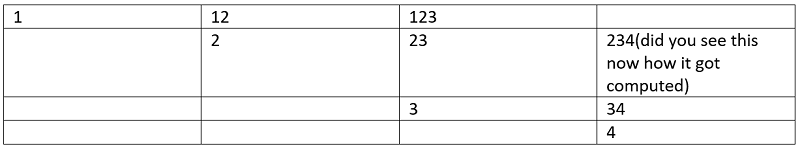Len=4

Start=0, end=3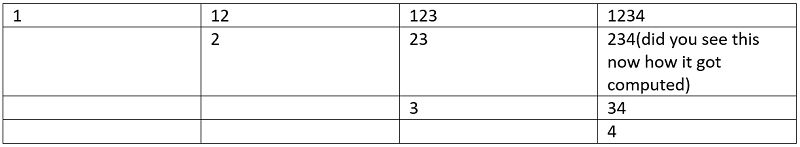At each step we have summed up, so result is stored at sum.

C++ Implementation:

``````#include <bits/stdc++.h>
using namespace std;

void print(vector<int> a, int n)
{
for (int i = 0; i < n; i++)
cout << a[i] << " ";
cout << endl;
}

long long int my(string s, int n)
{

long long int dp[n][n];
long long int sum = 0;
for (int i = 0; i < n; i++) {
dp[i][i] = s[i] - '0';
sum += dp[i][i];
}

for (int len = 2; len <= n; len++) {
for (int start = 0; start <= n - len; start++) {
int end = start + len - 1;
dp[start][end] = dp[start][end - 1] * 10 + s[end] - '0';
sum += dp[start][end];
}
}

return sum;
}
int main()
{
int t, n, item;

cout << "enter the string: ";
string s;
cin >> s;

cout << "sum of all possible substring is: " << my(s, s.length()) << endl;

return 0;
}
``````

Output:

```RUN 1:
enter the string: 17678
sum of all possible substring is: 29011

RUN 2:
enter the string: 326
sum of all possible substring is: 395```